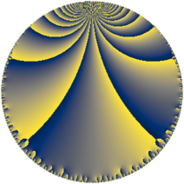# Properties

 Label 76.7.lLevel $76$ Weight $7$ Character orbit 76.l Rep. character $\chi_{76}(23,\cdot)$ Character field $\Q(\zeta_{18})$ Dimension $348$ Newform subspaces $1$ Sturm bound $70$ Trace bound $0$

# Related objects

## Defining parameters

 Level: $$N$$ $$=$$ $$76 = 2^{2} \cdot 19$$ Weight: $$k$$ $$=$$ $$7$$ Character orbit: $$[\chi]$$ $$=$$ 76.l (of order $$18$$ and degree $$6$$) Character conductor: $$\operatorname{cond}(\chi)$$ $$=$$ $$76$$ Character field: $$\Q(\zeta_{18})$$ Newform subspaces: $$1$$ Sturm bound: $$70$$ Trace bound: $$0$$

## Dimensions

The following table gives the dimensions of various subspaces of $$M_{7}(76, [\chi])$$.

Total New Old
Modular forms 372 372 0
Cusp forms 348 348 0
Eisenstein series 24 24 0

## Trace form

 $$348q - 6q^{2} + 192q^{4} - 12q^{5} - 492q^{6} - 3q^{8} + 1368q^{9} + O(q^{10})$$ $$348q - 6q^{2} + 192q^{4} - 12q^{5} - 492q^{6} - 3q^{8} + 1368q^{9} + 2811q^{10} - 3q^{12} - 7572q^{13} + 8217q^{14} - 1392q^{16} - 12q^{17} - 12q^{18} + 33618q^{20} - 33546q^{21} - 198q^{22} - 7128q^{24} - 12q^{25} + 84453q^{26} + 13464q^{28} - 12q^{29} + 75510q^{30} + 9339q^{32} - 366q^{33} - 289248q^{34} - 419349q^{36} - 24q^{37} + 158040q^{38} + 264570q^{40} + 27360q^{41} + 206001q^{42} - 525639q^{44} - 6q^{45} - 269328q^{46} - 703179q^{48} + 2319360q^{49} + 264534q^{50} + 130809q^{52} - 182292q^{53} + 607083q^{54} - 705906q^{56} - 12q^{57} - 710004q^{58} + 1060416q^{60} - 325092q^{61} - 1967868q^{62} - 1611921q^{64} + 398994q^{65} - 2295684q^{66} + 1125642q^{68} - 1625190q^{69} + 3496059q^{70} + 4948626q^{72} - 2224812q^{73} + 2661159q^{74} - 968262q^{76} + 974412q^{77} - 340743q^{78} - 3996663q^{80} + 4277754q^{81} - 3214221q^{82} - 872109q^{84} - 3207900q^{85} + 3189360q^{86} + 827973q^{88} - 2016084q^{89} - 710406q^{90} - 2545116q^{92} + 4486254q^{93} - 14322630q^{94} + 2623530q^{96} - 1223112q^{97} + 10989009q^{98} + O(q^{100})$$

## Decomposition of $$S_{7}^{\mathrm{new}}(76, [\chi])$$ into newform subspaces

Label Dim. $$A$$ Field CM Traces $q$-expansion
$$a_2$$ $$a_3$$ $$a_5$$ $$a_7$$
76.7.l.a $$348$$ $$17.484$$ None $$-6$$ $$0$$ $$-12$$ $$0$$